Next: Motion in a Nearly Up: Planetary Motion Previous: Kepler Problem

Motion in a General Central Force-Field

Consider the motion of an object in a general (attractive) central force-field characterized by the potential energy per unit mass function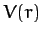. Since the force-field is central, it still remains true that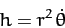(299)

is a constant of the motion. As is easily demonstrated, Equation (253) generalizes to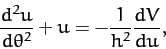(300)

where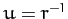.

Suppose, for instance, that we wish to find the potentialwhich causes an object to execute the spiral orbit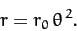(301)

Substitution of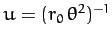into Equation (300) yields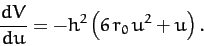(302)

Integrating, we obtain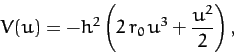(303)

or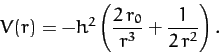(304)

In other words, the spiral pattern (301) is obtained from a mixture of an inverse-square and inverse-cube potential.Next: Motion in a Nearly Up: Planetary Motion Previous: Kepler Problem
Richard Fitzpatrick 2011-03-31